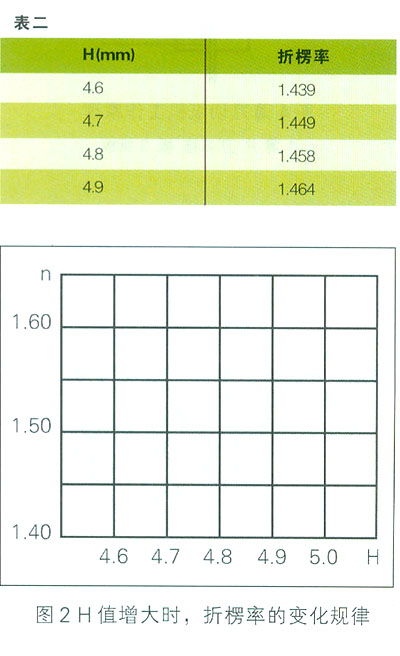# Base paper-based cost reduction program for corrugated board production (2)

The influence of the variation of tooth height H on the discount rate

For A corrugated cardboard, according to the relevant calculations, we obtain the relationship between tooth height and plication rate as shown in Table 2. According to Table 2, we can draw the curve of tooth height and plication rate as shown in Fig. 2, from Fig. 2. The curve can be seen that when the tooth height increases, the impact on the fold rate is also greater.When the tooth height H increases from 4.6 to 4.7, it can be found from Table 2 that the fold rate increases. 010
When the tooth height H increased from 4.6 to 4.8, from Table 2 can be found: the plication rate increased 0 019;
When the tooth height H increases from 4.6 to 4.9, it can be found from Table 2 that the fold rate increases. 025.
In this way, if the production of 5 million m2 single corrugated cardboard increased the amount of raw paper, respectively;
When H increases from 4.6 to 4.7, the increment of base paper is 0.010 x 5 x 106 = 50,000 m2
When H increases from 4.6 to 4.8, the increment of base paper is O.019 x 5 x 106 = 95,000 m2
When H increases from 4.6 to 4.9, the increment of base paper is 0.025 x 5 x 106 = 12.5 m2.

In other words: These base papers are consumed in vain, and the cost of paperboard is correspondingly increased. We know that the consumption of raw paper accounts for about 80% of the cost of carton production. We can simply calculate how much the original paper loss increases if the corrugation roll height increases from H = 4.6mm to H = 4.9mm for a cardboard line with a width of 1.6m. | Assume that the production speed V = 80m/min, according to 8 hours a day, the amount of production for one day.

L=1.6X 80 X 60 X 8-61440m2

Corrugated paper g=125g/m2=O.125kg/m2; annual basis for 310 days, the wasted paper is:
61440m2x 310 x 0.125 x 0.025 (increased discount rate) = 59,500 kg = 59.5 tons

That is, the corrugated board produced by a single-faced machine consumes 59.5 tons of core paper. This is only calculated based on 8h/day work. If it is a production of five-layer corrugated cardboard, two single-sided machines are needed. If you work 16 hours a day, the original paper that is lost is even more tolerable.

Of course, the above is just a brief description of high changes. In practice, the five-layer paperboards of two single-sided machines are not necessarily A-frames, and should be calculated according to the actual height of the corrugated roller roll, plus the Others. The impact of the parameter change, the consumption is considerable. Therefore, we must consider the size of the buckling rate when designing corrugated roll rams, which directly affects the economic efficiency of the company.

From the above analysis. We can conclude that reasonable choice of corrugation roll type parameters can effectively reduce the amount of raw paper and reduce production costs.

(to be continued)

Pest Control Series

Pest Control Series, Ultrasonic Pest Mice Rats Repeller Pest Control, China Pest Control Series

Mosquito Trap,Animal Repeller Co., Ltd. , http://www.chinapestrepeller.com